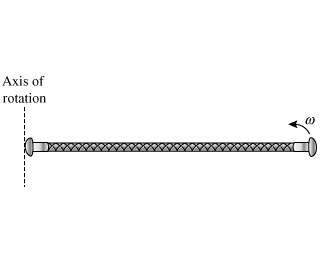# A majorette in a parade is performing some acrobatic twirlingsof her baton. Assume that the baton is a uniform rod of mass 0.120 kg and length 80.0 cmAnish Buchanan 2020-12-30 Answered

A majorette in a parade is performing some acrobatic twirlingsof her baton. Assume that the baton is a uniform rod of mass 0.120 kg and length 80.0 cm.
With a skillful move, the majorette changes the rotation ofher baton so that now it is spinning about an axis passing throughits end at the same angular velocity 3.00 rad/s as before. What is the new angularmomentum of the rod?

You can still ask an expert for help

• Live experts 24/7
• Questions are typically answered in as fast as 30 minutes
• Personalized clear answers

Solve your problem for the price of one coffee

• Math expert for every subject
• Pay only if we can solve itMaciej MorrowMoment of Inertia of the above baton $I=\left(\frac{1}{12}\right)\cdot M\cdot {L}^{2}$
M=0.120 kg
L=0.8 m
angular momentum of the rod $=I\omega$
$\omega$ is the angular speed = 3.00 rad/s